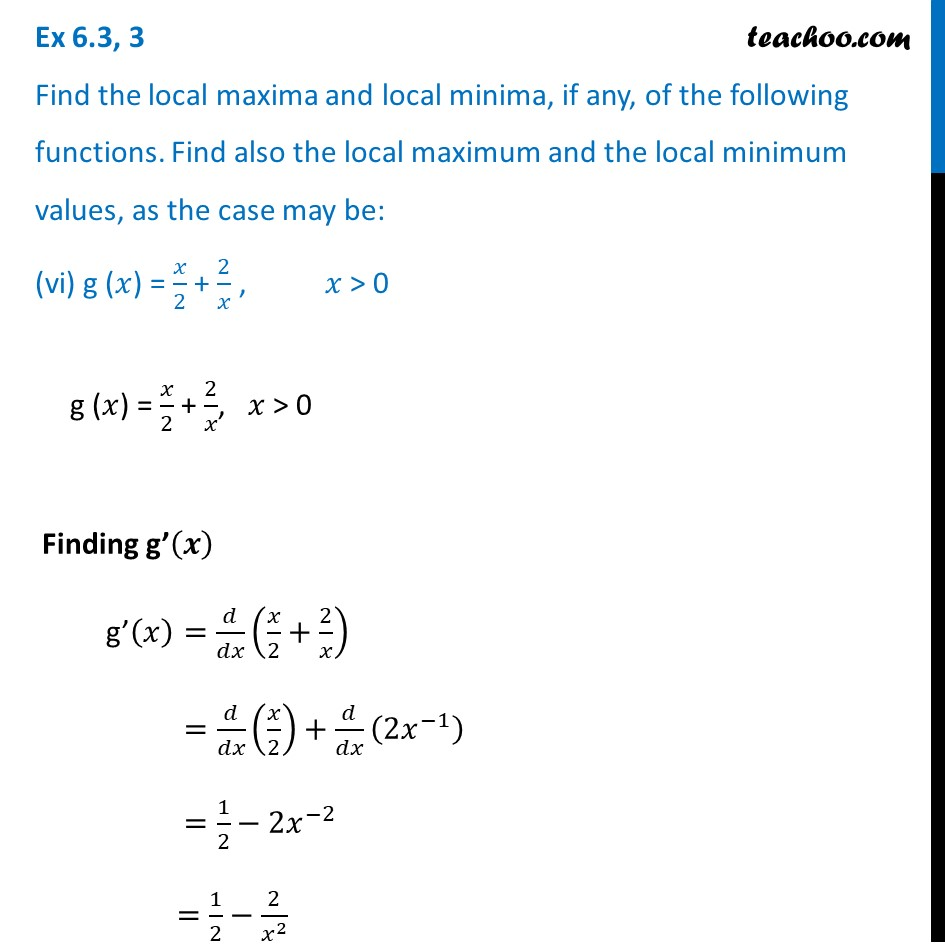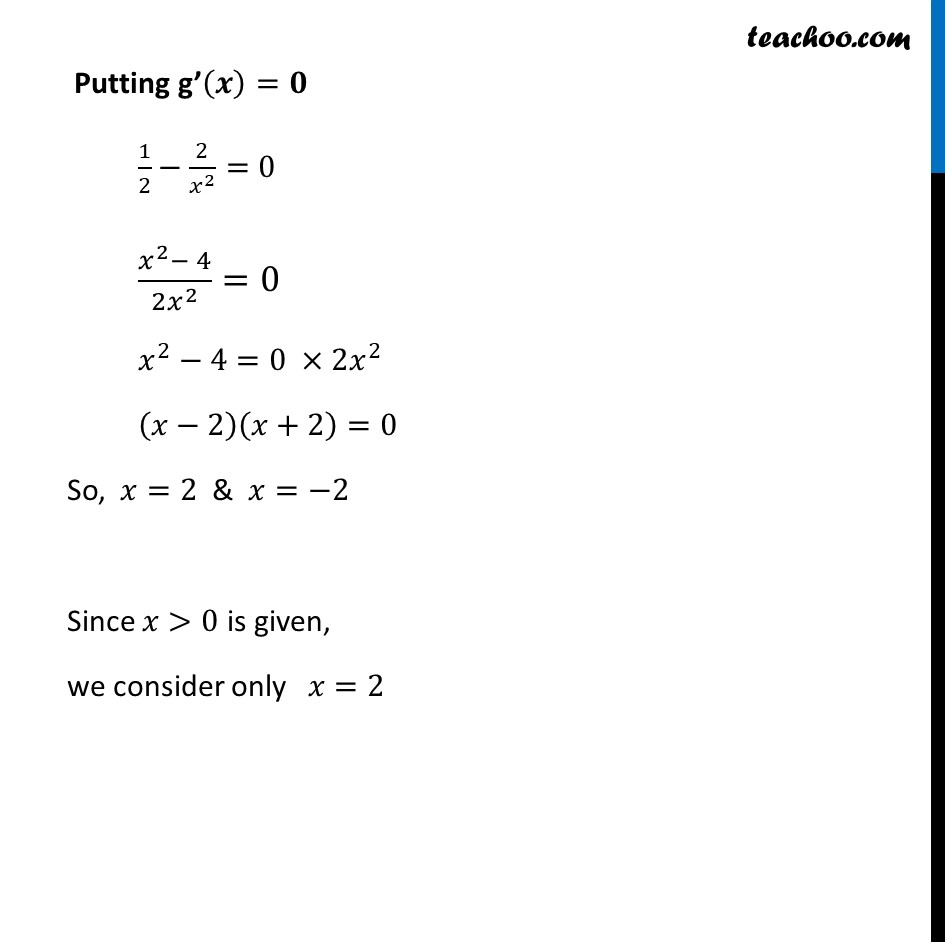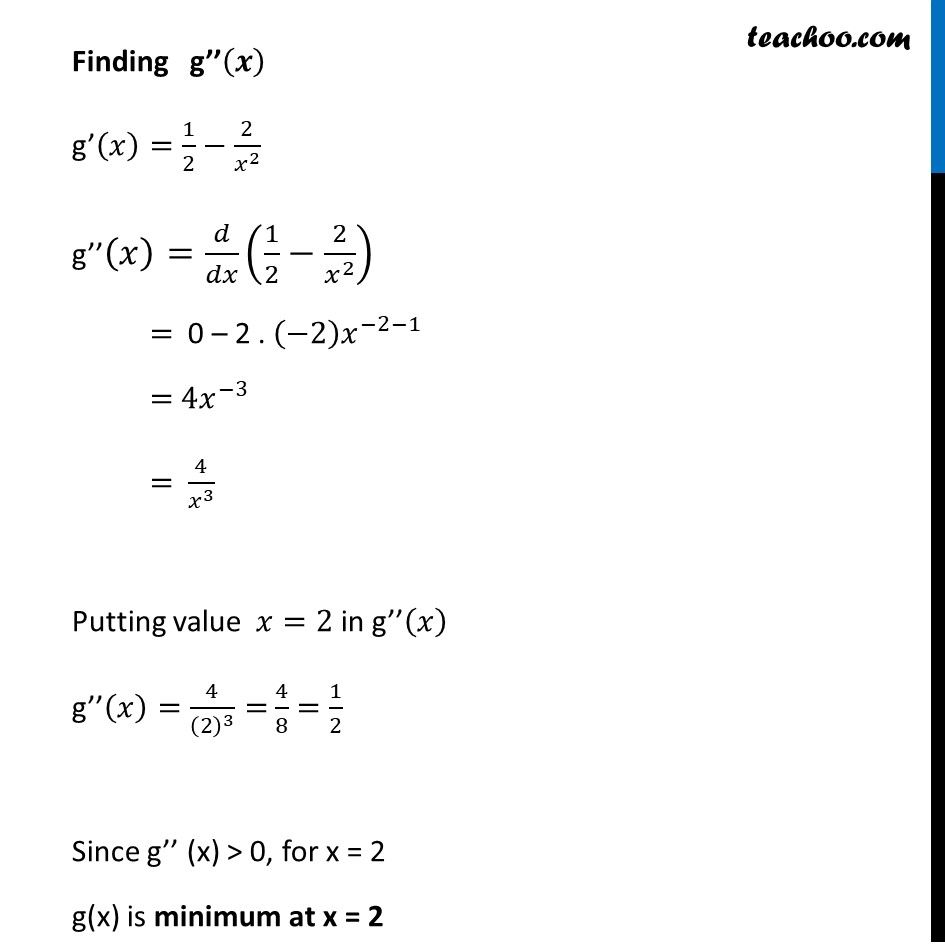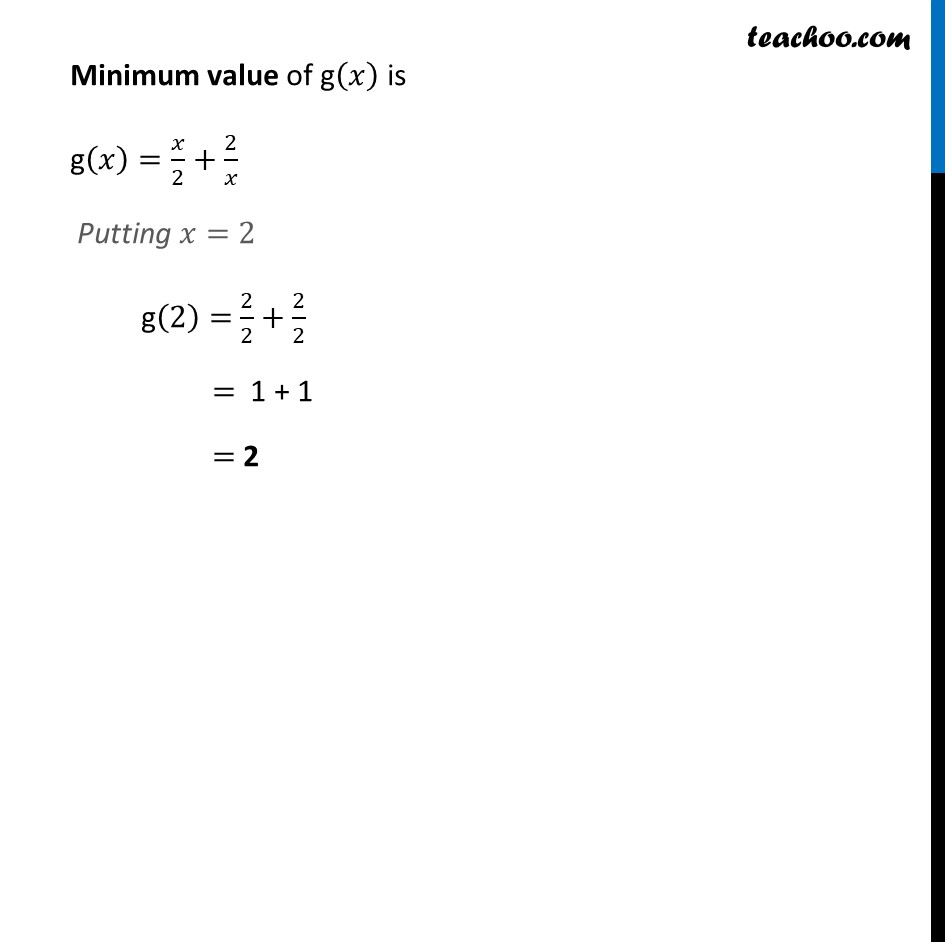Ex 6.3

Chapter 6 Class 12 Application of Derivatives
Serial order wiseLearn in your speed, with individual attention - Teachoo Maths 1-on-1 Class

### Transcript

Ex 6.3, 3 Find the local maxima and local minima, if any, of the following functions. Find also the local maximum and the local minimum values, as the case may be: (vi) g (𝑥) = 𝑥/2 + 2/𝑥 , 𝑥 > 0g (𝑥) = 𝑥/2 + 2/𝑥, 𝑥 > 0 Finding g’(𝒙) g’(𝑥)=𝑑/𝑑𝑥 (𝑥/2+2/𝑥) =𝑑/𝑑𝑥 (𝑥/2)+𝑑/𝑑𝑥 (2𝑥^(−1) ) =1/2−2𝑥^(−2) =1/2−2/𝑥^2 Putting g’(𝒙)=𝟎 1/2−2/𝑥^2 =0 (𝑥^2− 4)/(2𝑥^2 )=0 𝑥^2−4=0 ×2𝑥^2 (𝑥−2)(𝑥+2)=0 So, 𝑥=2 & 𝑥=−2 Since 𝑥>0 is given, we consider only 𝑥=2 Finding g’’(𝒙) g’(𝑥)=1/2−2/𝑥^2 g’’(𝑥)=𝑑/𝑑𝑥 (1/2−2/𝑥^2 ) = 0 – 2 . (−2) 𝑥^(−2−1) = 4𝑥^(−3) = 4/𝑥^3 Putting value 𝑥=2 in g’’(𝑥) g’’(𝑥)=4/(2)^3 = 4/8= 1/2 Since g’’ (x) > 0, for x = 2 g(x) is minimum at x = 2 Minimum value of g(𝑥) is g(𝑥)=𝑥/2+2/𝑥 Putting 𝑥=2 g(2)= 2/2+2/2 = 1 + 1 = 2